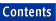Apache C++ Standard Library Reference Guide

## lower_bound()

Library:  Algorithms

`Function`

No Entries

### Summary

Algorithm that determines the first valid position for an element in a sorted container

### Synopsis

```#include <algorithm>

namespace std {
template <class ForwardIterator, class T>
ForwardIterator lower_bound(ForwardIterator start,
ForwardIterator finish,
const T& value);

template <class ForwardIterator, class T, class Compare>
ForwardIterator lower_bound(ForwardIterator start,
ForwardIterator finish,
const T& value,
Compare comp);
}
```

### Description

The lower_bound() algorithm compares a supplied value to elements in a sorted sequence and returns the first position in the container at which value can be inserted without violating the container's ordering. There are two versions of the algorithm. The first uses operator<() to perform the comparison, and assumes that the sequence has been sorted using that operator. The second version lets you include a function object of type Compare, and assumes that comp is the function used to sort the sequence. The function object must be a binary predicate.

The return value of lower_bound() is the iterator for the first element in the container that is greater than or equal to value, or, when the comparison operator is used, the first element that does not satisfy the comparison function. Formally, the algorithm returns an iterator i in the range [start, finish) such that for any iterator j in the range [start, i) the following corresponding conditions hold:

*j < value

or

comp(*j, value) == true

### Complexity

lower_bound() performs at most log(finish - start) + 1 comparisons.

### Example

```//
//  ul_bound.cpp
//

#include <vector>
#include <algorithm>
#include <functional>
#include <iostream>

int main ()
{
typedef std::vector<int, std::allocator<int> > Vector;
typedef Vector::const_iterator                 Iterator;

const int a[] = { 0, 1, 2, 2, 3, 4, 5, 5, 5, 6, 7 };

// Set up a vector.
const Vector v (a, a + sizeof a / sizeof *a);

// Try lower_bound variants.
Iterator it1 = std::lower_bound (v.begin (),v.end (), 3);
Iterator it2 = std::lower_bound (v.begin (),v.end (), 2,
std::less<int>());

// Try upper_bound variants.
Iterator it3 = std::upper_bound (v.begin (), v.end (), 3);
Iterator it4 = std::upper_bound (v.begin (), v.end (), 2,
std::less<int>());

std::cout << "\n\nThe upper and lower bounds of 3: ( "
<< *it1 << " , " << *it3
<< " ]\n\n\nThe upper and lower bounds of 2: ( "
<< *it2 << " , " << *it4 << " ]\n";

return 0;
}

Program Output:
```
```
The upper and lower bounds of 3: ( 3 , 4 ]

The upper and lower bounds of 2: ( 2 , 3 ]
```